Next: , Previous: Circular arcs, Up: GO Creating Modes

5.3.4.4 Elliptic arcs

The ellipse is just a special case of an elliptic arc; it can be changed into an elliptic arc just by changing the “conic” property. The “conic” property is quite important and very useful. It controls the type of the arc. The available values depend on the object type. The values for all types are:

• start-entire – a closed arc
• start-dif – an arc defined by the “start” parameter with “dif” defining the arc length

The available values for arcs with at least one point on the perimeter:

• point-entire – a closed arc
• point-dif – an arc starting from the start point with “dif” defining the arc length

The available values for arcs with three points on the perimeter:

• ccw – an arc connecting the three given points counterclockwise
• cw – an arc connecting the three given points clockwise
• smaller – the smaller one of the two arcs connecting the start point and the end point and having the third point on the perimeter of the whole circle/ellipse
• bigger – the larger one (dtto)
• middle – the arc connecting the start point and the end point via the center point
• opposite – the opposite to “middle”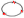The smallest ellipse defined by three points

Creates the smallest (in area) ellipse determined by three points on its perimeter. The points must not be collinear.

Type: elliptic-arc
Subtype: ellipse-by-3-points-smallest
Hangers: curve, start, end, center
Anchors: point-1, point-2, point-3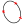Ellipse defined by three points, rotation and eccentricity

Creates an ellipse defined by three points on its perimeter, the rotation and eccentricity. The points must not be collinear. The rotation and eccentricity properties can be edited in the Property editor.

Type: elliptic-arc
Subtype: ellipse-by-3-points-rotation-eccentricity
Hangers: curve, start, end, center
Anchors: point-1, point-2, point-3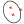Ellipse defined by two foci and a point

Creates an ellipse defined by the foci and a point on its perimeter. The two foci points must not be equal.

Type: elliptic-arc
Subtype: ellipse-by-2-foci-point
Hangers: curve, start, end, center
Anchors: focus-1, focus-2, point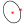Ellipse defined by the center point, a point and eccentricity

Creates an ellipse defined by the center point, a point on its perimeter and eccentricity. The eccentricity property can be edited in the Property editor.

Type: elliptic-arc
Subtype: ellipse-by-center-point-rotation-eccentricity
Hangers: curve, start, end, center
Anchors: center, point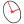Ellipse defined by the center point and radii

Creates an ellipse defined by the center point and the two radii. The radii can be edited in the Property editor.

Type: elliptic-arc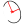Elliptic arc defined by the center point and radii